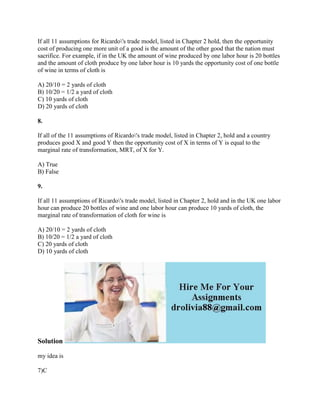Seu SlideShare está sendo baixado. ×

# If all 11 assumptions for Ricardo-'s trade model- listed in Chapter 2.docx

Anúncio
Anúncio
Anúncio
Anúncio
Anúncio
Anúncio
Anúncio
Anúncio
Anúncio
Anúncio
AnúncioCarregando em…3
×

1 de 2 Anúncio

# If all 11 assumptions for Ricardo-'s trade model- listed in Chapter 2.docx

If all 11 assumptions for Ricardo\'s trade model, listed in Chapter 2 hold, then the opportunity cost of producing one more unit of a good is the amount of the other good that the nation must sacrifice. For example, if in the UK the amount of wine produced by one labor hour is 20 bottles and the amount of cloth produce by one labor hour is 10 yards the opportunity cost of one bottle of wine in terms of cloth is
A) 20/10 = 2 yards of cloth
B) 10/20 = 1/2 a yard of cloth
C) 10 yards of cloth
D) 20 yards of cloth
8.
If all of the 11 assumptions of Ricardo\'s trade model, listed in Chapter 2, hold and a country produces good X and good Y then the opportunity cost of X in terms of Y is equal to the marginal rate of transformation, MRT, of X for Y.
A) True
B) False
9.
If all 11 assumptions of Ricardo\'s trade model, listed in Chapter 2, hold and in the UK one labor hour can produce 20 bottles of wine and one labor hour can produce 10 yards of cloth, the marginal rate of transformation of cloth for wine is
A) 20/10 = 2 yards of cloth
B) 10/20 = 1/2 a yard of cloth
C) 20 yards of cloth
D) 10 yards of cloth
Solution
my idea is
7)C
8)A
9)B
.

If all 11 assumptions for Ricardo\'s trade model, listed in Chapter 2 hold, then the opportunity cost of producing one more unit of a good is the amount of the other good that the nation must sacrifice. For example, if in the UK the amount of wine produced by one labor hour is 20 bottles and the amount of cloth produce by one labor hour is 10 yards the opportunity cost of one bottle of wine in terms of cloth is
A) 20/10 = 2 yards of cloth
B) 10/20 = 1/2 a yard of cloth
C) 10 yards of cloth
D) 20 yards of cloth
8.
If all of the 11 assumptions of Ricardo\'s trade model, listed in Chapter 2, hold and a country produces good X and good Y then the opportunity cost of X in terms of Y is equal to the marginal rate of transformation, MRT, of X for Y.
A) True
B) False
9.
If all 11 assumptions of Ricardo\'s trade model, listed in Chapter 2, hold and in the UK one labor hour can produce 20 bottles of wine and one labor hour can produce 10 yards of cloth, the marginal rate of transformation of cloth for wine is
A) 20/10 = 2 yards of cloth
B) 10/20 = 1/2 a yard of cloth
C) 20 yards of cloth
D) 10 yards of cloth
Solution
my idea is
7)C
8)A
9)B
.

Anúncio
Anúncio

### If all 11 assumptions for Ricardo-'s trade model- listed in Chapter 2.docx

1. 1. If all 11 assumptions for Ricardo's trade model, listed in Chapter 2 hold, then the opportunity cost of producing one more unit of a good is the amount of the other good that the nation must sacrifice. For example, if in the UK the amount of wine produced by one labor hour is 20 bottles and the amount of cloth produce by one labor hour is 10 yards the opportunity cost of one bottle of wine in terms of cloth is A) 20/10 = 2 yards of cloth B) 10/20 = 1/2 a yard of cloth C) 10 yards of cloth D) 20 yards of cloth 8. If all of the 11 assumptions of Ricardo's trade model, listed in Chapter 2, hold and a country produces good X and good Y then the opportunity cost of X in terms of Y is equal to the marginal rate of transformation, MRT, of X for Y. A) True B) False 9. If all 11 assumptions of Ricardo's trade model, listed in Chapter 2, hold and in the UK one labor hour can produce 20 bottles of wine and one labor hour can produce 10 yards of cloth, the marginal rate of transformation of cloth for wine is A) 20/10 = 2 yards of cloth B) 10/20 = 1/2 a yard of cloth C) 20 yards of cloth D) 10 yards of cloth Solution my idea is 7)C
2. 2. 8)A 9)B